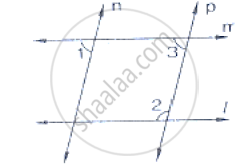# In the Below Fig, If L || M, N || P and ∠1 = 85°, Find ∠2. - Mathematics

In the below fig, if l || m, n || p and ∠1 = 85°, find ∠2.#### Solution

Corresponding angles are equal

∠1 = ∠3 = 85°

By using the property of co-interior angles are supplementary

∠2 + ∠3 = 180°

∠2 + 55° = 180°

∠2 = 180° - 55°

∠2 = 95°

∴ ∠2 = 95°

Concept: Pairs of Angles
Is there an error in this question or solution?

#### APPEARS IN

RD Sharma Mathematics for Class 9
Chapter 10 Lines and Angles
Exercise 10.4 | Q 5 | Page 47